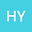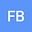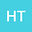Method of distributions for quantification of geologic uncertainty in flow simulations
•••• +1
Hyung Jun Yang
Stanford University
Author ProfileHamdi A. Tchelepi
Stanford University
Author Profile## Abstract

Probabilistic models of subsurface flow and transport are required for risk assessment and reliable decision making under uncertainty. These applications require accurate estimates of confidence intervals, which generally cannot be ascertained with such statistical moments as mean (unbiased estimate) and variance (a measure of uncertainty) of a quantity of interest (QoI). The method of distributions provides this information by computing either the probability density function or the cumulative distribution functions (CDF) of the QoI. The method can be orders of magnitude faster than Monte Carlo simulations (MCS), but is applicable to stationary, mildly-to-moderately heterogeneous porous media in which the coefficient of variation of input parameters (e.g., log-conductivity) is below three. Our CDF-RDD framework alleviates these limitations by combining the method of distributions and the random domain decomposition (RDD); it also accounts for uncertainty in the geologic makeup of a subsurface environment. For a given realization of the geological map, we derive a deterministic equation for the conditional CDF of hydraulic head of steady single-phase flow. The solutions of this equation are then averaged over realizations of the geological maps to compute the hydraulic head CDF. Our numerical experiments reveal that the CDF-RDD remains accurate for two-dimensional flow in a porous material composed of two heterogeneous hydrofacies, a setting in which the original CDF method fails. For the same accuracy, the CDF-RDD is an order of magnitude faster than MCS.